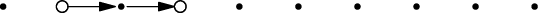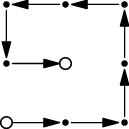시간 제한메모리 제한제출정답맞힌 사람정답 비율
1 초 1024 MB0000.000%

## 문제

You are given a rectangular grid consisting of r × c points. The lower left corner has coordinates (1,1), the upper right corner has coordinates (c,r). The neighbors of a point (x,y) are the points (x − 1,y), (x + 1,y), (x,y − 1), and (x,y + 1), if they exist. A path is a sequence of points such that subsequent points are neighbors and each point appears on the path at most once.

Given two distinct points (xs,ys) and (xf,yf), ﬁnd one longest path from (xs,ys) to (xf,yf).

## 입력

The ﬁrst line of the input ﬁle contains an integer t specifying the number of test cases. Each test case is preceded by a blank line.

Each test case consists of a single line with six integers – r, c, xs, ys, xf, yf.

You may assume that 1 ≤ r,c ≤ 100 and rc ≥ 2.

## 출력

For each test case, output a single string describing one possible longest path. If there are multiple longest paths, output any one of them.

A path a1,a2,…,ak is described by a string consisting of k − 1 letters U, D, L, R. The i-th letter in the string describes the move from point ai to ai+1:

• If ai = (x,y) and ai+1 = (x,y + 1), the i-th letter should be U.
• If ai = (x,y) and ai+1 = (x,y − 1), the i-th letter should be D.
• If ai = (x,y) and ai+1 = (x + 1,y), the i-th letter should be R.
• If ai = (x,y) and ai+1 = (x − 1,y), the i-th letter should be L.

번호배점제한
Easy1

r ≤ 5.

Hard2

## 예제 입력 1

2

1 10 2 1 4 1

3 3 1 1 2 2


## 예제 출력 1

RR
RRUULLDR


## 힌트

ﬁrst example:second example:## 채점 및 기타 정보

• 예제는 채점하지 않는다.